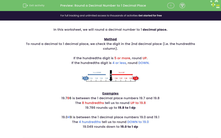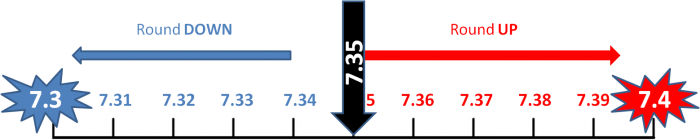# Round a Decimal Number to 1 Decimal Place

In this worksheet, students will round decimal numbers to 1 decimal place.Key stage:  KS 3

Curriculum topic:   Number

Curriculum subtopic:   Round/Approximate Numbers Appropriately

Popular topics:   Rounding worksheets

Difficulty level:#### Worksheet Overview

In this activity, we will round a decimal number to 1 decimal place.

Method

To round a decimal to 1 decimal place, we check the digit in the 2nd decimal place (i.e. the hundredths column).

If the hundredths digit is 5 or more, round UP.

If the hundredths digit is 4 or less, round DOWN.Examples

19.786 is between the 1 decimal place numbers 19.7 and 19.8

The 8 hundredths tell us to round UP to 19.8

19.786 rounds up to 19.8 to 1 dp

19.049 is between the 1 decimal place numbers 19.0 and 19.1

The 4 hundredths tell us to round DOWN to 19.0

19.049 rounds down to 19.0 to 1 dp

Does that make sense?Let's get started!

### What is EdPlace?

We're your National Curriculum aligned online education content provider helping each child succeed in English, maths and science from year 1 to GCSE. With an EdPlace account you’ll be able to track and measure progress, helping each child achieve their best. We build confidence and attainment by personalising each child’s learning at a level that suits them.

Get started